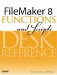# PMT()

Category: Financial

 Syntax: PMT ( principal; interestRate; term )

Parameters:

principalA number (or numeric expression) representing the initial amount borrowed.

interestRateA decimal number (or numeric expression), representing the monthly interest rate used to amortize the principal amount. Given an annual interest rate, you can divide by 12 to get the monthly interest rate.

termA number (or numeric expression) representing the period of loan, expressed in months.

Data type returned: Number

Description:

Returns the monthly payment that would be required to pay off the principal based on the interestRate and term specified.

The PMT calculation makes it easy to see the effect of interest rates on the monthly payment of an installment loan. For instance, buying a \$20,000 car at 6.9% for 48 months would require a \$478.00 monthly payment, but at 3.9%, the payment would be \$450.68.

Examples:

Someone borrows \$1,000 from you, and you want to set up a payment schedule whereby the loan is paid off in 2 years at an interest rate of 6%. To determine the monthly payment,

``` PMT (1000; .06/12; 24)
```

returns \$44.32.FileMaker 8 Functions and Scripts Desk Reference
ISBN: 0789735113
EAN: 2147483647
Year: 2004
Pages: 352